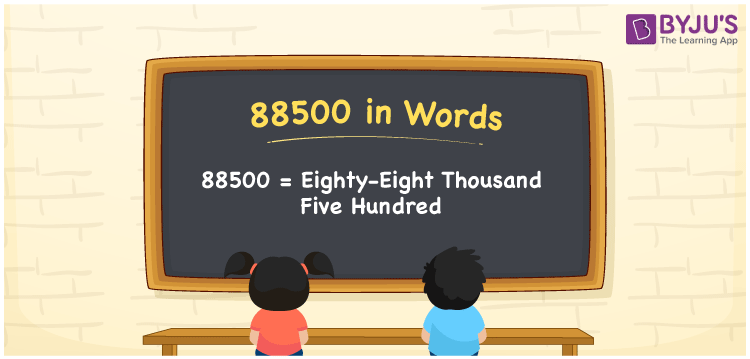# 88500 in Words

88500 in words is written as “Eighty-eight thousand five hundred”. The number 88500 contains five digits and it is a cardinal number. For example, Arjun has saved Rs. 88500 in 9 months. This article discusses the procedure of writing the number 88500 in words using the place value system.

 88500 in Words: Eighty-eight Thousand Five Hundred. Eighty-eight Thousand Five Hundred in Numerical Form: 88500.

## 88500 in English Words## How to Write 88500 in Words?

Learn the place values of 88500 using the below table.

 Ten-thousands Thousands Hundreds Tens Ones 8 8 5 0 0

The expanded form of 88500 is as follows:

= 8 × Ten thousand + 8 × Thousand + 5 × Hundred + 0 × Ten + 0 × One

= 8 × 10000 + 8 × 1000 + 5 × 100 + 0 × 10 + 0 × 1

= 80000 + 8000 + 500

= 88500

= Eighty-eight thousand five hundred

Hence, 88500 in words is eighty-eight thousand five hundred.

88500 in words – Eighty-eight thousand five hundred

Is 88500 an odd number? – No

Is 88500 an even number? – Yes

Is 88500 a perfect square number? – No

Is 88500 a perfect cube number? – No

Is 88500 a prime number? – No

Is 88500 a composite number? – Yes

## Frequently Asked Questions on 88500 in Words

Q1

### Write 88500 in words.

88500 in words is eighty-eight thousand five hundred.

Q2

### Simplify 88000 + 500, and express it in words.

Simplifying 88000 + 500, we get 88500. Hence, 88500 in words is eighty-eight thousand five hundred.

Q3

### Is 88500 a prime number?

No, 88500 is not a prime number.